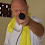## Saturday, January 14, 2017

### QUANTUM MATHEMATICS FOR QUANTUM REALITY

This is the abstract for a paper I'm preparing for a mathematical physics journal. Obviously it is a technical and mathematical paper, but I plan to post it here after it has been published.

QUANTUM MATHEMATICS FOR QUANTUM REALITY
By Edward R. Close, PhD, DSPE
ABSTRACT
Arguably the most important scientific discovery in modern times is the revelation that we exist in a quantized reality governed by the laws of general relativity. By far the most successful mathematical procedure used by scientists to analyze physical reality is the calculus of Newton and Leibniz. But, mainly because of its successes, the fact that Newtonian calculus is mathematically inappropriate for application to the quantum phenomena revealed by Planck and Einstein discoveries has been largely overlooked. It is the theme of this paper that this oversight causes much of the co-called “quantum weirdness” that physicists often talk about, and that the proper analysis of a quantized reality requires an appropriately quantized system of mathematical logic.

In this paper, focusing on the four most basic elementary particles: photons, electrons, up-quarks and down-quarks, a system of mathematical logic operating on quantum mass-energy-volumetric equivalence units is introduced. The Triadic Rotational Unit of Equivalence (TRUE) quantum equivalence unit is derived from the basic principles of quantum mechanics, relativity and particle-wave complementarity. Introduction of quantum equivalence units enables us to revise the calculus of Newton and Leibniz to produce a calculus that is more appropriate for application to the phenomena of relativistic quantum reality.

Applying this new calculus to elementary particles and combinations of elementary particles, we obtain a clearer understanding of the sub-atomic, atomic and molecular structure of reality. The use of this system of quantized mathematical logic clears up much of the “quantum weirdness”, yields new information about the multi-dimensional nature of reality, and makes the scientific description and analysis of quantum phenomena much more comprehensible and complete. As a result, experimental data that seemed irrelevant become meaningful, and some observations that are inexplicable in the current paradigm, are explained.

1.In the further interest of the Ultimate Force's simple 'Y (Universal Intelligence)= X (Elements 0,1,2,3,4) Squared plus One' formula, engrafted into my mind during my 1980 mystical-initiation, I feel your explanation below about the generalized mathematical-symbolism related to this article, and my comments on your Facebook homepage site are worth repeating here for the sake of posterity - They follow:

Brian Walker: Lost in the symbolism, Ed, but I'm glad it equals One! IJN!

Edward R. Close: This is the general expression for the combination of elementary particles forming the atoms of reality. It represents an infinity of combinations, but the one you'll like is: When n = m = 2, and X sub 2 = 1, it yields X squared plus 1.

Brian Walker: Thanks, Ed - You've made my and the Ultimate Force's day! IJN!

What next, I have to wonder?

2.Dr Close, You should find Sweet Sue at a one of her many threads re; cancer,hope,gratuity,gentleness, revelation today. Please don't be put off by the site "420 mag" as inspired thought Is there. We seek Truth , not enlightenment , Truth has no rules. Peace BE With You ALWAYS my friend Joy To The World PS She Is pleasant-attractive-effortless Peace again always

3.Silence is an illusion Awareness has made. A shelter away from perpetual change. Within itself it can watch itself fade, into nothing that will never change. You are not alone Living in change, We are all One Being, Slightly rearranged. walleye Peace 1-25-17

4.John 7;18 7;26

5.The speed of light is inertial resistance. The following law explains both E=mc2 AND F=ma. Gravitational force/energy is proportional (or balanced with/as) inertia/inertial resistance, as this balances and unifies gravity AND ELECTROMAGNETISM/energy. This is the very greatest law/truth in all of physics, by far. Accordingly, gravity/acceleration involves balanced inertia/inertial resistance; as ELECTROMAGNETISM/energy is gravity. So, a given planet sweeps out equal areas in equal times.

Outer "space" involves full inertia, AND it is fully invisible AND black.

By Frank DiMeglio

6.The speed of light is inertial resistance. The following law explains both E=mc2 AND F=ma. Gravitational force/energy is proportional (or balanced with/as) inertia/inertial resistance, as this balances and unifies gravity AND ELECTROMAGNETISM/energy. This is the very greatest law/truth in all of physics, by far. Accordingly, gravity/acceleration involves balanced inertia/inertial resistance; as ELECTROMAGNETISM/energy is gravity. So, a given planet sweeps out equal areas in equal times.

Outer "space" involves full inertia, AND it is fully invisible AND black.

By Frank DiMeglio